# 4 2 Standard Form of a Quadratic Function

• Slides: 194. 2 – Standard Form of a Quadratic Function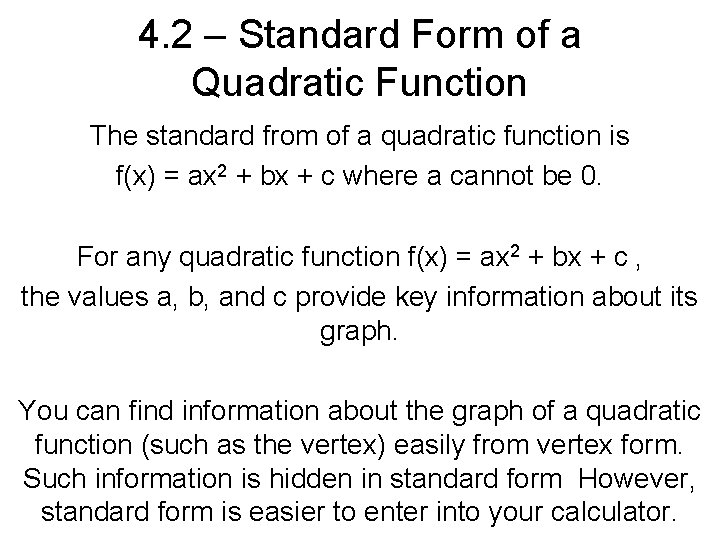4. 2 – Standard Form of a Quadratic Function The standard from of a quadratic function is f(x) = ax 2 + bx + c where a cannot be 0. For any quadratic function f(x) = ax 2 + bx + c , the values a, b, and c provide key information about its graph. You can find information about the graph of a quadratic function (such as the vertex) easily from vertex form. Such information is hidden in standard form However, standard form is easier to enter into your calculator.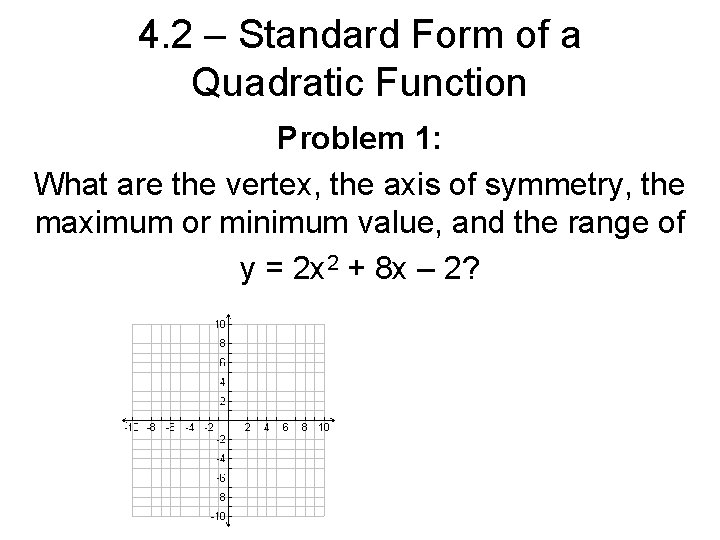4. 2 – Standard Form of a Quadratic Function Problem 1: What are the vertex, the axis of symmetry, the maximum or minimum value, and the range of y = 2 x 2 + 8 x – 2?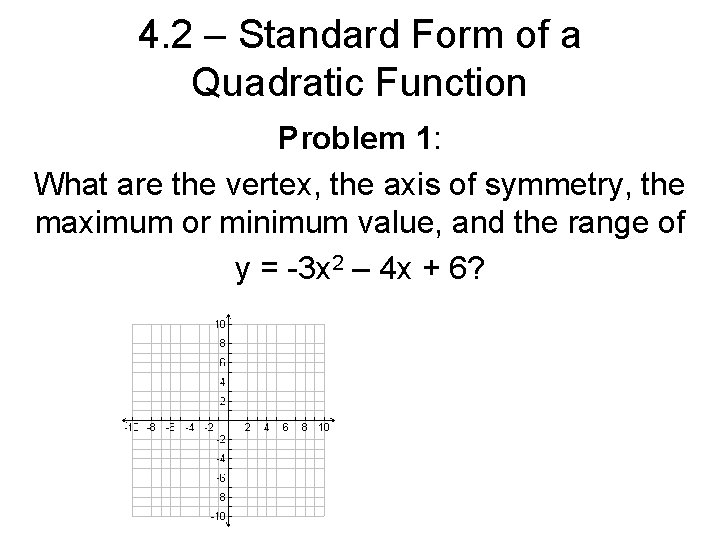4. 2 – Standard Form of a Quadratic Function Problem 1: What are the vertex, the axis of symmetry, the maximum or minimum value, and the range of y = -3 x 2 – 4 x + 6?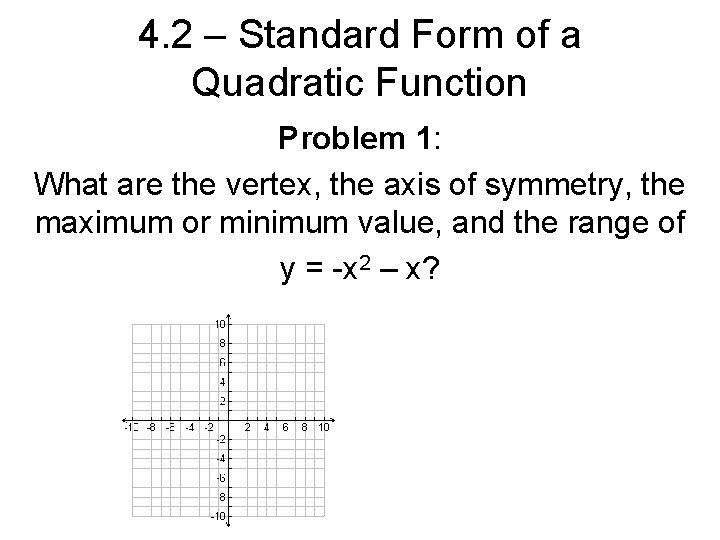4. 2 – Standard Form of a Quadratic Function Problem 1: What are the vertex, the axis of symmetry, the maximum or minimum value, and the range of y = -x 2 – x?4. 2 – Standard Form of a Quadratic Function4. 2 – Standard Form of a Quadratic Function Problem 2: What is the graph of y = x 2 + 2 x + 3?4. 2 – Standard Form of a Quadratic Function Problem 2: What is the graph of y = -2 x 2 + 2 x – 5?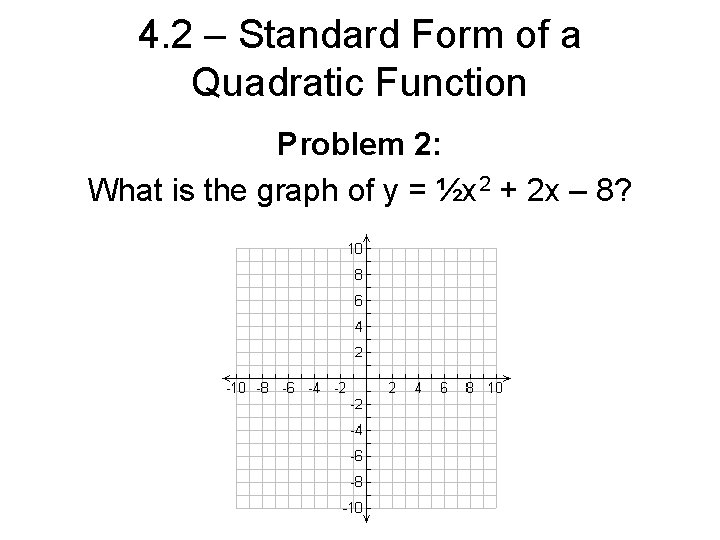4. 2 – Standard Form of a Quadratic Function Problem 2: What is the graph of y = ½x 2 + 2 x – 8?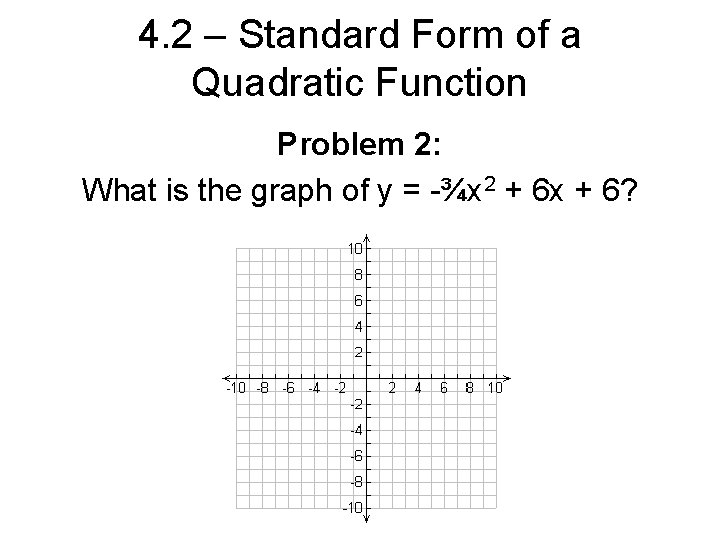4. 2 – Standard Form of a Quadratic Function Problem 2: What is the graph of y = -¾x 2 + 6 x + 6?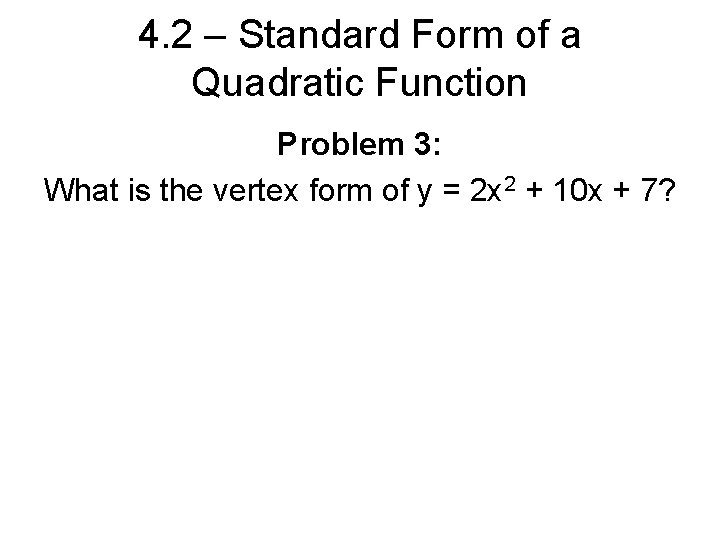4. 2 – Standard Form of a Quadratic Function Problem 3: What is the vertex form of y = 2 x 2 + 10 x + 7?4. 2 – Standard Form of a Quadratic Function Problem 3: What is the vertex form of y = -x 2 + 4 x – 5 ?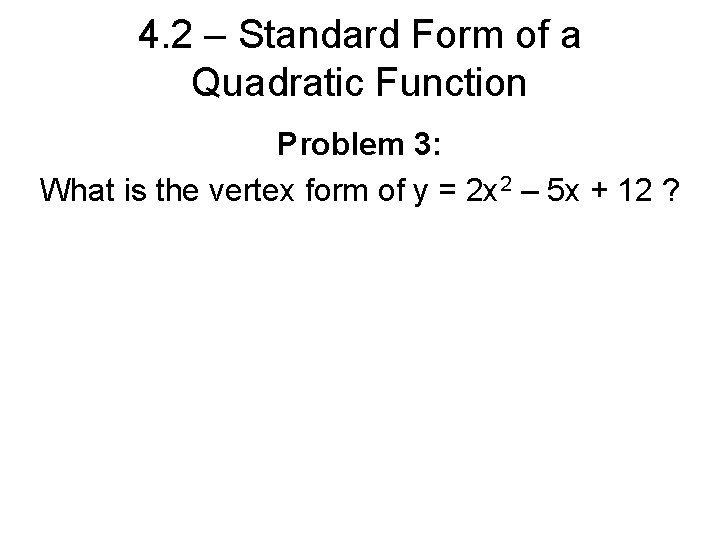4. 2 – Standard Form of a Quadratic Function Problem 3: What is the vertex form of y = 2 x 2 – 5 x + 12 ?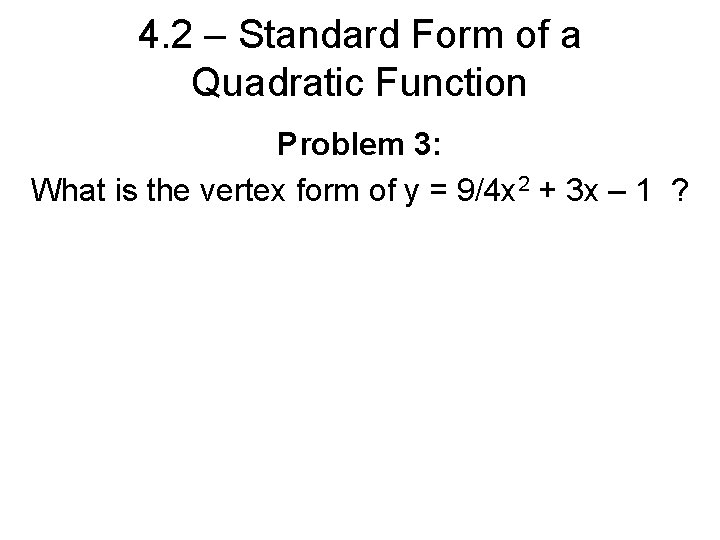4. 2 – Standard Form of a Quadratic Function Problem 3: What is the vertex form of y = 9/4 x 2 + 3 x – 1 ?4. 2 – Standard Form of a Quadratic Function Problem 4: For each function, find the y-intercept: y = (x – 1)2 + 2 ?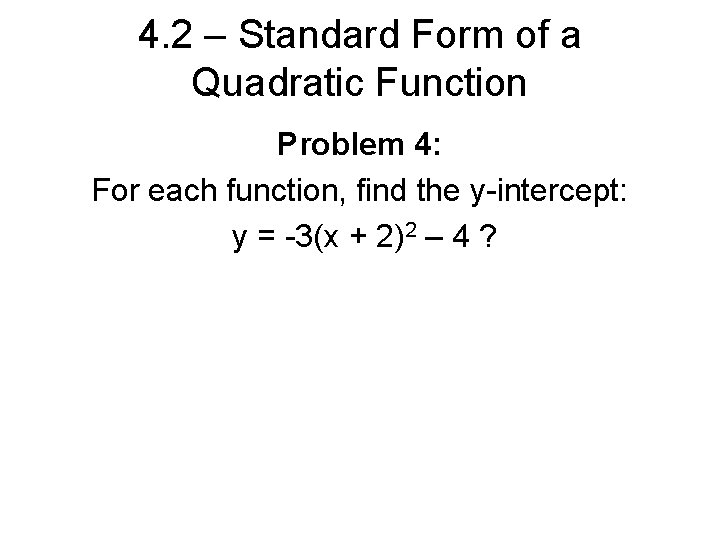4. 2 – Standard Form of a Quadratic Function Problem 4: For each function, find the y-intercept: y = -3(x + 2)2 – 4 ?4. 2 – Standard Form of a Quadratic Function The New River Gorge Bridge in West Virginia is the world’s largest steel single arch bridge. You can model the arch with the function y = -0. 000498 x 2 + 0. 847 x, where x and y are in feet. How high above the river is the arch? How long is the section of bridge above the arch?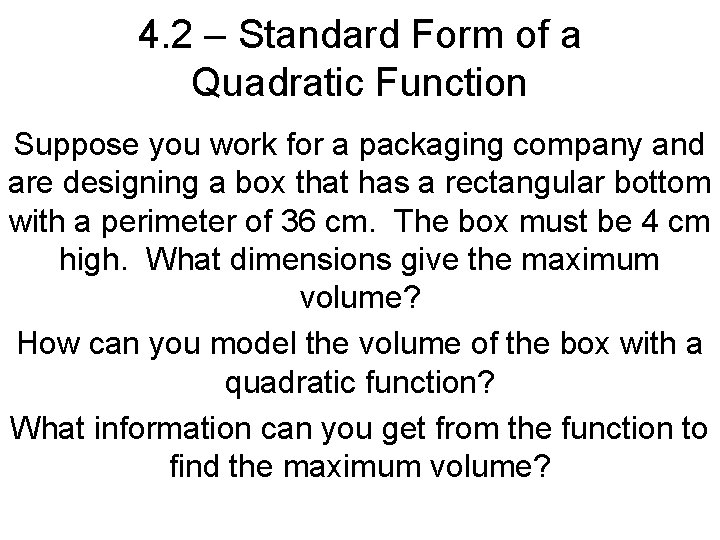4. 2 – Standard Form of a Quadratic Function Suppose you work for a packaging company and are designing a box that has a rectangular bottom with a perimeter of 36 cm. The box must be 4 cm high. What dimensions give the maximum volume? How can you model the volume of the box with a quadratic function? What information can you get from the function to find the maximum volume?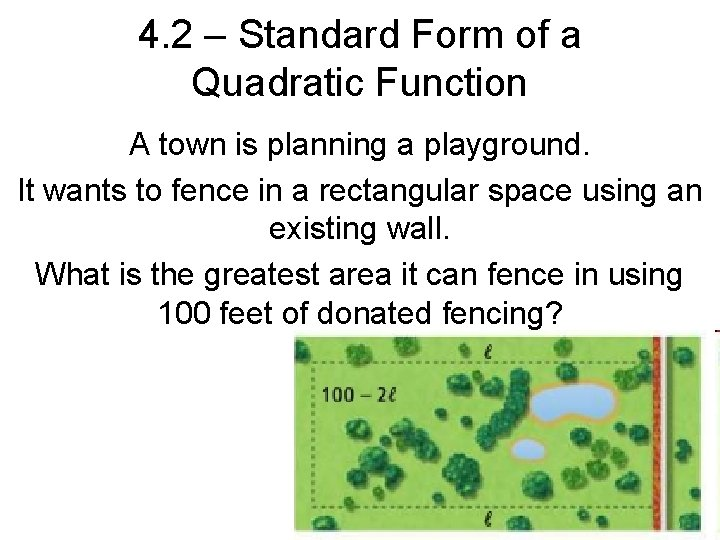4. 2 – Standard Form of a Quadratic Function A town is planning a playground. It wants to fence in a rectangular space using an existing wall. What is the greatest area it can fence in using 100 feet of donated fencing?